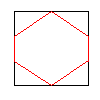# Touch INSIDE a Hexagon

I have a hexagon (drawn inside a rectange)it is easy to determine if an XY coor is inside the rectangle, but what is the math to determine if it is only inside the Hexagon (note it will be a true hexagon, this image is just an example)

given the rectangle is X,Y,W,H in size (where W and H are always equal)

1 Like

one lazy way is to color the interior then test if x,y is that color

but you knew that one and I’m sure threw it out as “not super useful”I’ll assume a “regular” hexagon ?
there’s a few answers on SO ( in JAVA !!! OMG !)

there’s a much more general one for just about any polygonal shape here

Thanks to both of you… I should be able to work it out from all thatThis explains the in my eyes best mathematical wa to find a solution.

And yes, from my side and not Java @npalardy

Are you feeling OK ?just kidding

Nas when waking up in the morning I hear all time such weird sounds of silence and there is a kind of feeling cold and I have sand in my mouth… probably I have also Problems that I become green…maybe something’s not okayOut drinking and fell asleep on the beach ?Hope you feel better soon

I feel like in a hexagon cage…

don’t let yourself be touched …

Here a Xojo class for here made by Eugene Dakin

Thanks… that might help… don’t use Xojo anymore, but the logic should work

1. Calculate the side length (s) of the hexagon: Since the width and height of the rectangle are equal (W = H), the side length of the hexagon can be calculated as follows: s = W / (1 + 2 * cos(30°))
2. Determine the height (h) of the hexagon: h = s * sqrt(3) / 2
3. Calculate the coordinates of the hexagon’s vertices. Let the top-left corner of the rectangle be the origin (0, 0). The hexagon’s vertices can be calculated as follows: A (0.5 * s, 0)
4. B (1.5 * s, 0)
5. C (2 * s, h)
6. D (1.5 * s, 2 * h)
7. E (0.5 * s, 2 * h)
8. F (0, h)
9. Check if the point (x, y) is inside the hexagon by dividing the hexagon into six triangles, formed by connecting each pair of consecutive vertices to the center of the hexagon (O). The coordinates of the center can be calculated as: O (s, h)
10. For each triangle (AOB, BOC, COD, DOE, EOF, FOA), check if the point (x, y) is inside the triangle using the following method:
• Calculate the area of the triangle using the coordinates of its vertices.
• Calculate the area of the three triangles formed by the point (x, y) and each pair of consecutive vertices of the triangle.
• If the sum of the areas of the three triangles is equal to the area of the original triangle, the point (x, y) is inside the triangle.
11. If the point (x, y) is inside any of the six triangles, it is inside the hexagon. Otherwise, it is outside the hexagon.
1 Like